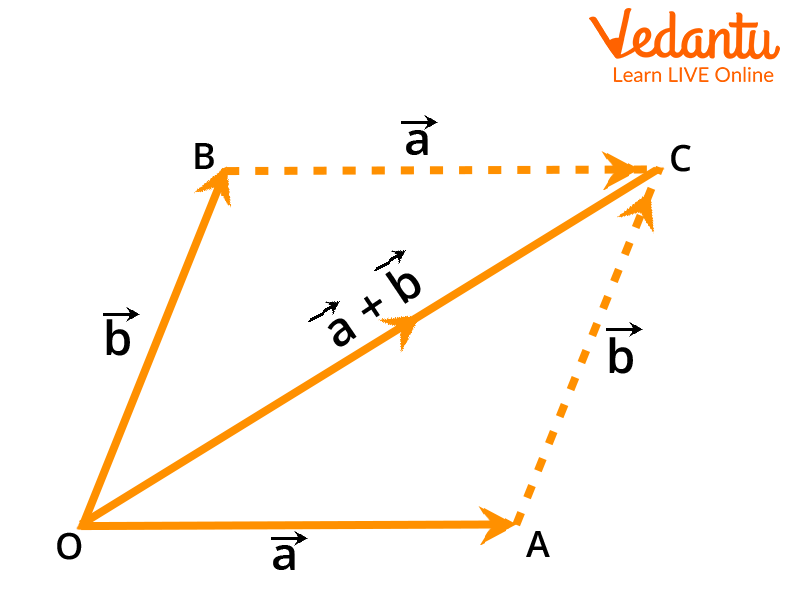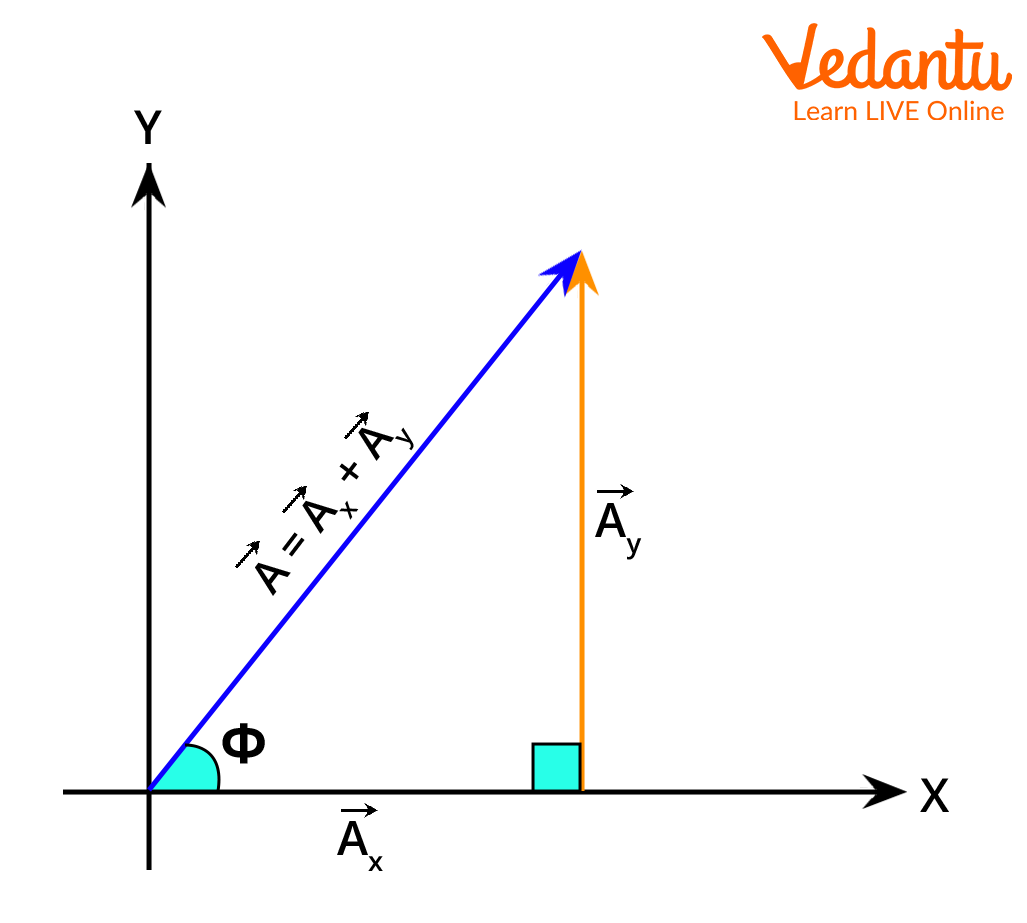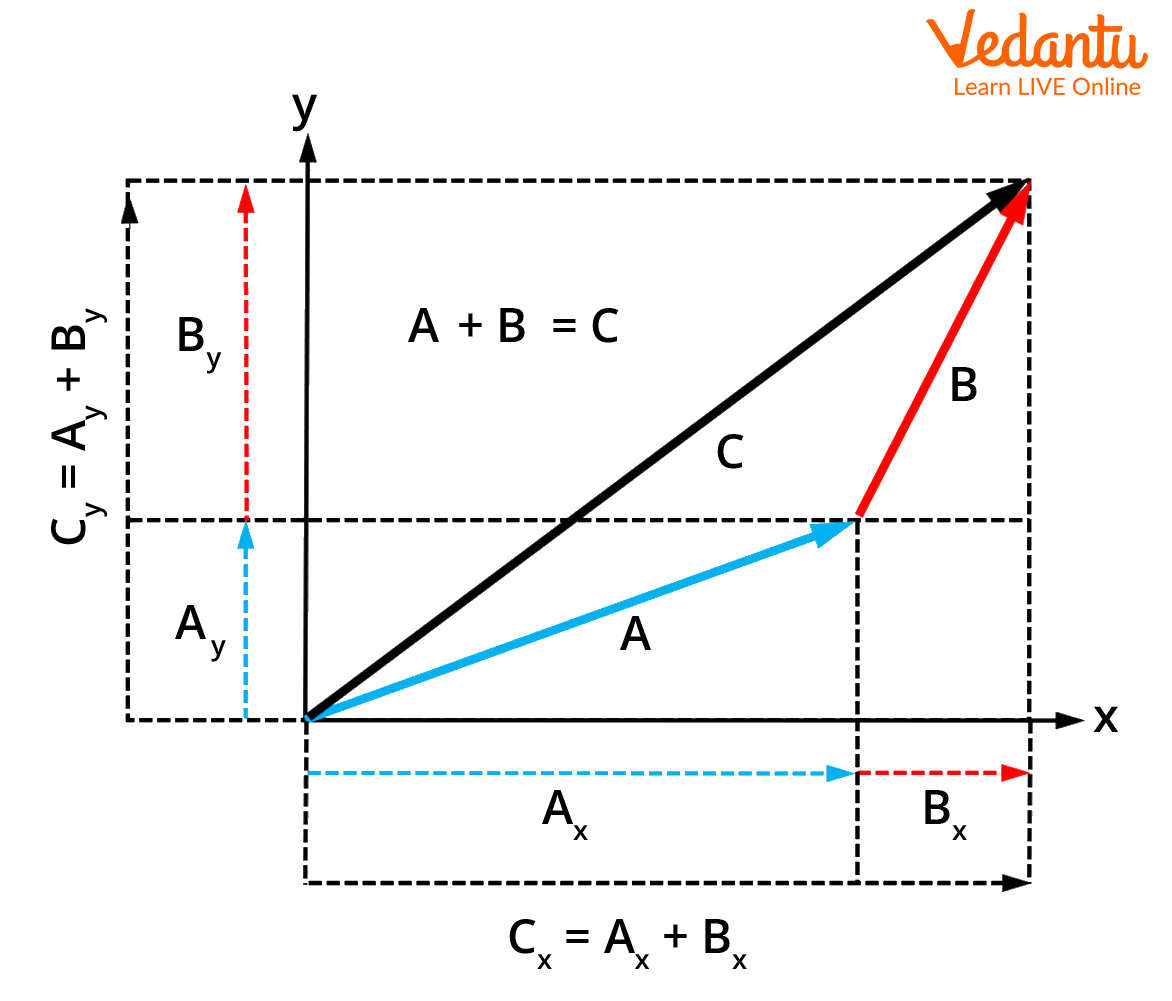Courses
Courses for Kids
Free study material
Free LIVE classes
More

# Analytical Method of Vector Addition | Important Topic for JEELIVE
Join Vedantu’s FREE Mastercalss

Adding two or more vectors is known as vector addition. While adding vectors, we use the addition operation to combine two or more vectors to create a new vector that is equal to the total of the vectors. Vector addition is used in the algebra of physical quantities where velocity, displacement, and acceleration are represented by vectors. Solving equations from Newton’s second law of motion also requires the use of vectors where the forces are represented by vectors. The total force on a body is the vector sum of all the forces acting on that body. Not only in mechanics, but vectors also find their use in almost all branches of physics, and vector addition is one of the most basic operations on vectors. Its knowledge is absolutely crucial for solving physics problems.

Since vectors have both a direction and a magnitude, the algebra performed on them is also different from the typical scalar algebra. They have their own properties, and they have to be considered whenever vector addition is being performed. The different properties of vector addition have been listed below.

### 1. Existence of an Identity

We say that an identity exists for any vector because for any vector a, we can write,

a+0=a

In the above equation, we can say that the 0 vector is the additive identity of the vector a.

### 2. Existence of an Inverse

This property states that there exists an additive inverse for any vector. This means that if we have a vector a then its inverse -a exists, and it satisfies,

a+(-a)=0

### 3. Commutativity

The order of vector addition for any two vectors does not matter, as the final result is the same no matter the order in which the vectors are added. Suppose we have vectors a and b. Then for their addition, the order won’t matter. We can write this mathematically as,

a+b=b+a

### 4. Associativity

The addition of three vectors is unaffected by the pair which is added first. The order of the addition used for the three vectors does not matter, and the final answer is the same. Suppose, we have three vectors a, b, c, then the associativity can be written as,

(a+b)+c=a+(b+c)

## Different Ways of Vector Addition

Vectors can be added in different ways and these methods are graphical in nature as the vectors have a direction that is to be considered. The methods involved in vector addition involve a mixture of graphical and mathematical methods. We’ll just list the methods and will go into detail only for the analytical method of vector addition.

The methods of vector addition are:

### Triangle Law of Vector Addition

The triangle law of vector addition is also known as the head-to-tail method. According to this method, two vectors can be joined together by arranging them so that the first vector's head meets the tail of the second vector. We can acquire the resultant vector sum by linking the tail of the first vector to the head of the second vector. The diagram below will explain this law more clearly. In the diagram, we have two vectors M and N and their resultant is represented by S.### Parallelogram Law of Vector Addition

This law states that if the two neighbouring sides (both in magnitude and direction) of a parallelogram formed from a point can represent two vectors, then the resultant sum vector can be totally represented by the diagonal of the parallelogram drawn from the same point.

Suppose we have two vectors a and b, then their addition using the parallelogram law of vector addition is given in the diagram below.## Analytical Method of Vector Addition

The ruler and protractor of graphical methods are replaced by geometry and basic trigonometry in the analytical methods of vector addition and subtraction. In order to make vectors easier to visualise, arrows are still included as a part of the graphical technique. However, compared to graphical approaches, which are constrained by the precision of a drawing, analytical methods are more succinct, exact, and precise. The precision and accuracy with which physical quantities are known is the only restriction on analytical approaches.

Suppose we have a vector in the Cartesian plane, then we can decompose the vector into its vertical and horizontal components. We can then add these components of one vector individually to their corresponding components of another vector. This will give the addition of those two vectors. This concept will become clear with an example.Breaking the vector into components

From the above diagram, we can see that the vector A has been decomposed into its x and y components which are represented by Ax and Ay respectively. It is evident from the diagram that the three vectors form a triangle, and we can write the vector A as the sum of the other two components. This means that,

$A_{x}+A_{y}=A$

The values of Ax and Ay in terms of A can be written using trigonometry as,

\begin{align} &A_{x}=A \cos \phi \\ &A_{v}=A \sin \phi \end{align}

We can also write down the magnitude of the vector A as,

$\left|A^{2}\right|=\sqrt{A_{x}^{2}+A_{y}^{2}}$

We can write down the angle as,

$\phi=\tan ^{-1} \dfrac{A_{y}}{A_{x}}$

Thus, if we have any vector, we can find its magnitude and direction by breaking it down into components.

If we have to add two vectors, we can break the vectors down into components, add the components, and then find the magnitude and direction of the resultant vector. This will be more clear with an example. Suppose we have vectors A and B in a Cartesian plane as before. We will write the components for B as we have already written down the components of A. If we consider the angle that B makes with the x-axis as θ, then the components are:

\begin{align} &B_{x}=B \cos \theta \\ &B_{y}=B \sin \theta \end{align}We can then write the components of the resultant of A and B which we denote by C as,

\begin{align} &C_{x}=A_{x}+B_{x} \\ &C_{y}=A_{y}+B_{y} \end{align}

The magnitude of this vector C will be,

\begin{align} &|C|=\sqrt{C_{x}{ }^{2}+C_{y}{ }^{2}} \\ &|C|=\sqrt{\left(A_{x}+B_{x}\right)^{2}+\left(A_{y}+B_{y}\right)^{2}} \end{align}

Similarly, the direction will be,

\begin{align} &\alpha=\tan ^{-1}\left(\dfrac{C_{y}}{C_{x}}\right) \\ &\alpha=\tan ^{-1}\left(\frac{A_{y}+B_{y}}{A_{x}+B_{x}}\right) \end{align}

This concept will become more clear with the addition of vector examples.

## Numerical Examples of Analytical Method of Vector Addition

Example 1: A vector has a magnitude of 10 units, and it makes an angle of 30° with the x-axis. Similarly, another vector has a magnitude of 5 units, and it makes an angle of 60° with the x-axis. Both vectors are at the origin, so find the magnitude of the resultant of both vectors.

Solution:

We have A=10 and $\theta=30^{\circ}$. Similarly, B=5, and $\varphi=60^{\circ}$. We can write the components as,

\begin{align} &A_{x}=10 \cos 30^{\circ} \\ & \Rightarrow A_{x}=8.66 \\ &A_{y}=10 \sin 30^{\circ} \\ & \Rightarrow A_{y}=5 \\ & \Rightarrow B_{x}=5 \cos 60^{\circ} \\ & \Rightarrow B_{x}=2.5 \\ & \Rightarrow B_{y}=5 \sin 60^{\circ} \\ & \Rightarrow B_{x}=4.33 \end{align}

So we can write,

\begin{align} &C_{x}=8.66+2.5 \\ & \Rightarrow C_{x}=11.16 \\ & \Rightarrow C_{y}=5+4.33 \\ &C_{y}=9.33 \end{align}

The magnitude of C will be,

\begin{align} &|C|=\sqrt{(9.33)^{2}+(11.16)^{2}} \\ & \Rightarrow |C|=\sqrt{87.0489+124.5456} \\ & \therefore |C|=14.5456 \end{align}

So the magnitude of the resultant of the two given vectors is 14.5456 units.

Example 2: Find the direction of the resultant vector from example 1.

Solution: The x and y components of the resultant of example 1 are,

\begin{align} &C_{x}=11.16 \\ &C_{y}=9.33 \end{align}

The direction will then be,

\begin{align} &\alpha=\tan ^{-1}\left(\dfrac{C_{y}}{C_{x}}\right) \\ & \Rightarrow \alpha=\tan ^{-1}\left(\dfrac{9.33}{11.16}\right) \\ & \therefore \alpha=39.89^{\circ} \end{align}

The direction of the resultant vector from example 1 is 39.89°.

## Conclusion

Vector addition is extremely useful in physics because most physical quantities in physics can be represented by vectors and their addition is essential. Vector addition has certain properties that it follows, and since vectors have both a direction and magnitude, vectors have their own algebra. Vectors can be added using graphical methods. The methods that can be used are the triangle law of vector addition, parallelogram law of vector addition, and the analytical method of vector addition. The analytical method is precise, and it uses graphical methods and a bit of trigonometry. In the analytical method, we break down the vectors into components and then add the corresponding components to find the resultant.

Last updated date: 21st Sep 2023
Total views: 133.8k
Views today: 4.33k

## FAQs on Analytical Method of Vector Addition | Important Topic for JEE

1. What are some real-life applications of vectors?

Vectors are used to understand the force's attempt to move the body in a specific direction. To understand how gravity acts as an attracting force on a body. To compute a body's motion that is contained within a plane. They are also used to explain the simultaneous, three-dimensional force operating on a body (3-D form). In engineering, vectors are utilised when a force is greater than what a structure can withstand without collapsing.

2. What are coplanar and concurrent vectors?

Coplanar vectors are the vectors that lie in the same place. These vectors might be perpendicular to each other, but as long as they lie in the same plane, they are called coplanar. The vectors in the triangle law of vector addition are coplanar. The vectors that originate from the same point are known as concurrent vectors. One example of concurrent vectors could be the vectors that we use in the parallelogram law of vector addition. In this law, the vectors originate from the same point.